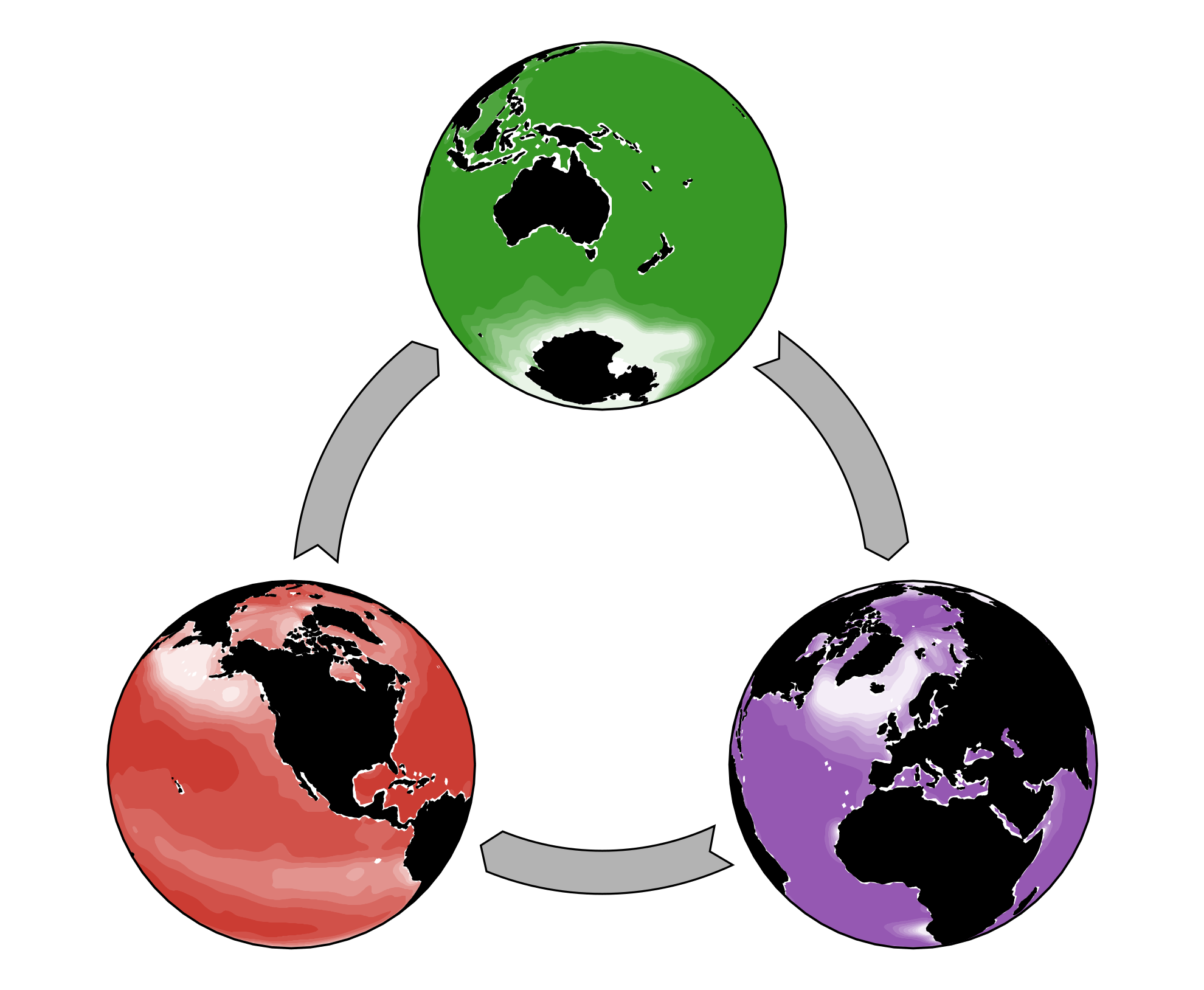## AIBECS.jl

The ideal tool for exploring global marine biogeochemical cycles.
Popularity
34 Stars
Updated Last
7 Months Ago
Started In
May 2019# AIBECS.jl

The ideal tool for exploring global marine biogeochemical cycles.

AIBECS (for Algebraic Implicit Biogeochemical Elemental Cycling System, pronounced like the cool ibex) is a Julia package that provides ocean biogeochemistry modellers with an easy-to-use interface for creating and running models of the ocean system.

AIBECS is a system because it allows you to choose some biogeochemical tracers, define their interactions, select an ocean circulation and Voilà! — your model is ready to run.

## Getting started

If you are new to AIBECS, head over to the documentation and look for the tutorials. (You can also click on the big "Documentation" badge above.)

## Concept

This package was developed to exploit linear-algebra tools and algorithms in Julia to efficiently simulate marine tracers. AIBECS represents global biogeochemical cycles with a discretized system of nonlinear ordinary differential equations that takes the generic form

$$\frac{∂\boldsymbol{x}}{∂t} + \mathbf{T} \boldsymbol{x} = \boldsymbol{G}(\boldsymbol{x})$$

where $\boldsymbol{x}$ represents the model state variables, i.e., the marine tracer(s) concentration. For a single tracer, $\boldsymbol{x}$ can be interpreted as the 3D field of its concentration. In AIBECS, $\boldsymbol{x}$ is represented as a column vector (that's why it's bold and italic).

The operator $\mathbf{T}$ is a spatial differential operator that represents the transport of tracers. For example, for a single tracer transported by ocean circulation,

$$\mathbf{T} = \nabla \cdot(\boldsymbol{u} - \mathbf{K}\nabla)$$

represents the effects of advection and eddy-diffusion (where $\boldsymbol{u}$ is the 3D vector of the marine currents and $\mathbf{K}$ is a 3×3 eddy-diffusivity matrix). Thus, $\mathbf{T}$ "acts" on $\boldsymbol{x}$ such that $\mathbf{T}\boldsymbol{x}$ is the flux divergence of that tracer. In AIBECS, $\mathbf{T}$ is represented by a matrix (that's why it's bold and upstraight).

Lastly, the right-hand-side, $\boldsymbol{G}(\boldsymbol{x}$), represents the local sources minus sinks of each tracer, which must be provided as functions of the tracer(s) $\boldsymbol{x}$.

To simulate tracers using the AIBECS, you just need to define the transport operators $\mathbf{T}$ and the net sources and sinks $\boldsymbol{G}$. That's pretty much the whole concept!

## References

If you use this package, please cite it.

If you use data provided by this package (like the ocean circulation from the OCIM), please cite them as well.

For convenience, all the references are available in BibTeX format in the CITATION.bib file.

Also, if you want to do research using the AIBECS, and you think I could help, do not hesitate to contact me directly (contacts on my website), I would be happy to contribute and collaborate!The authors acknowledge funding from the Department of Energy grant DE-SC0016539 and from the National Science Foundation grant 1658380.

### Required Packages

View all packages

### Used By Packages

No packages found.